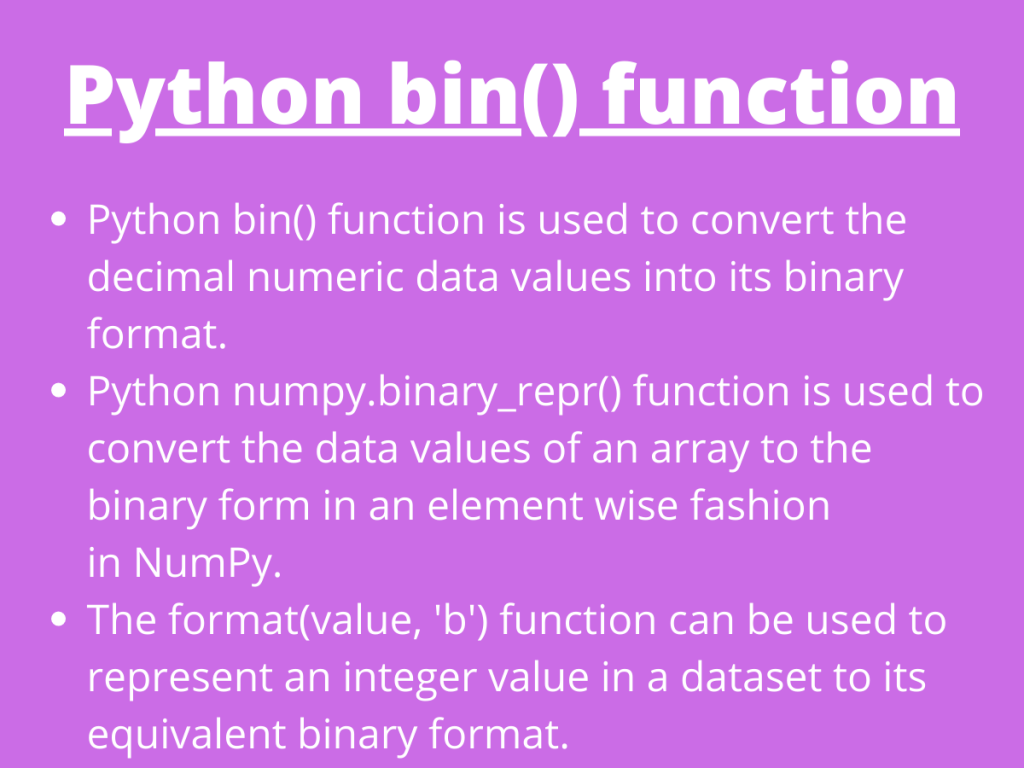# What is Python bin() function?Hey, folks! In this article, we will be focusing on the Binary representation of a data value using the Python bin() function.

## Getting started with Python bin() function

Python has various in-built functions to deal and perform manipulations on the numeric data.

Python `bin() function` is used to convert the decimal numeric data values into its binary format.

Syntax:

```bin(number)
```

The `bin() function` returns the binary representation value of the integer passed to it as an argument with a prefix ‘0b’ attached to it.

Example 1: Converting a positive numeric value to its binary form

```num = 3
res_bin = bin(num)
print(res_bin)
```

Output:

```0b11
```

Example 2: Converting a negative numeric value to its binary format

```num = -3
res_bin = bin(num)
print(res_bin)
```

Output:

```-0b11
```

## Binary representation of elements in NumPy

Python `numpy.binary_repr() function` is used to convert the data values of an array to the binary form in an element wise fashion in NumPy.

Syntax:

```numpy.binary_repr(array[value],width)
```
• `width`: This parameter defines the length of the returned string representing the binary format.
• If a negative value is passed to the function and width is not specified, then a minus(‘-‘) sign is added prior to the result. In case the width is specified, two’s complement of the number is represented as the absolute value.

Example 1:

```import numpy as N
arr = [3,5,6,2]
res_arr = N.binary_repr(arr)
print(res_arr)
```

Output:

```101
```

Example 2:

```import numpy as N
arr = [3,5,6,2]
res_arr = N.binary_repr(arr,width=5)
print(res_arr)
```

Output:

```00101
```

## Binary representation of data elements in Pandas

It is possible for us to represent the elements of the dataset in Pandas in a binary format. The format() function can be used to represent an integer value in a dataset to its equivalent binary format.

We can simply use the `apply() function` and create an anonymous function to imply the manipulation of every data value using Python lambda and format() function.

Syntax:

```data['column'].apply(lambda element: format(int(element), 'b'))
```

Dummy dataset:

Example:

```import pandas as PD
data_few = PD.DataFrame(data['custAge'].iloc[2:4])
data_few['custAge'].apply(lambda i: format(int(i), '05b'))
```

In the above piece of code, we have used the format(value, ‘b’) function to convert the data values into binary form. Further, we have created a function to achieve the same functionality using lambda expression. The ’05b’ represents the length of the return string i.e. length = 5.

Output:

```2    101010
3    110111
Name: custAge, dtype: object
```

## Conclusion

Thus, in this article, we have understood the way to represent the integer value to a binary form using the Python bin() function.

## References

• Python bin() function — JournalDev